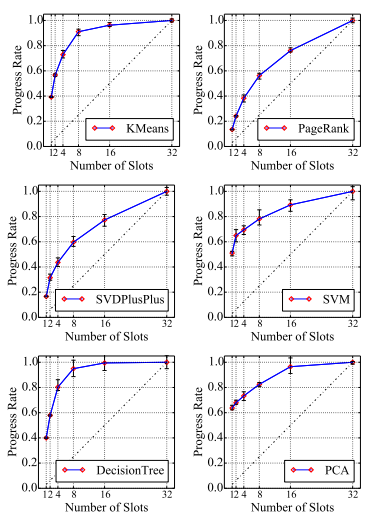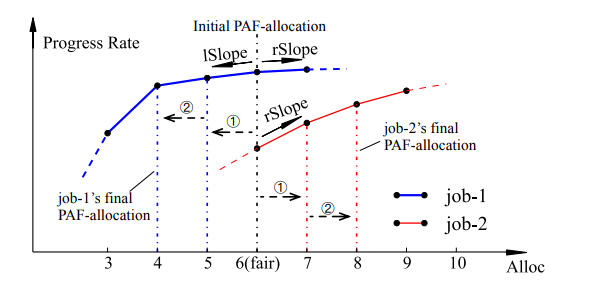PDF

# 现象

slot 数量和作业完成时间不是线性关系# 原因

2. JVM warming up

# 前提

1. 完全并行, 使用computer slot
2. 满载

# 定义

1. Job Completion Time (JCT)
2. Progress Rate = $Shortest\ JCT \over JCT\ with\ the\ allocated\ slots$

# 优化

$$\max_{ x\overrightarrow = (x_1,x_2,…,x_n) } \sum_i{p_i(x_i)}$$ $$subject\ to\ p_i(x_i) \ge a*p_i(f_i), i=1,…,n$$ 其中 $a$ 是一个叫做牺牲度的参数

# 实现# 结果

$a=0.9$ progress rate 从 0.78 到 0.99 (15%), $a=0.99$ 时仍有13%的提高

github